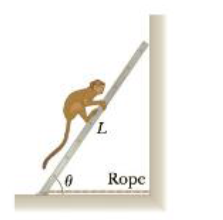Chapter 8, Problem 94AP

Chapter
Section
Textbook Problem

A 10.0-kg monkey climbs a uniform ladder with weight w = 1.20 x 102 N and length L = 3.00 m as shown in Figure P8.94. The ladder rests against the wall at an angle of θ = 60.0C. The upper and lower ends of the ladder rest on frictionless surfaces, with the lower end fastened to the wall by a horizontal rope that is frayed and that can support a maximum tension of only 80.0 N. (a) Draw a force diagram for the ladder, (b) Find the normal force exerted by the bottom of the ladder, (c) Find the tension in the rope when the monkey is two-thirds of the way up the ladder, (d) Find the maximum distance d that the monkey can climb up the ladder before the rope breaks, (e) If the horizontal surface were rough and the rope were removed, how would your analysis of the problem be changed and what other information would you need to answer parts (c) and (d)?Figure P8.94

(a)

To determine
Free body diagram for the ladder.

Explanation

The following figure shows the free body diagram.

(b)

To determine
The normal force exerted by the bottom of the ladder.

(c)

To determine
The tension in the rope when the monkey is two-thirds of the way up the ladder.

(d)

To determine
The maximum distance d that the monkey can climb up the ladder.

(e)

To determine
The required alternate analysis if the rope would be removed and the surface be enough rough.

Still sussing out bartleby?

Check out a sample textbook solution.

See a sample solution

The Solution to Your Study Problems

Bartleby provides explanations to thousands of textbook problems written by our experts, many with advanced degrees!

Get Started

Find more solutions based on key concepts